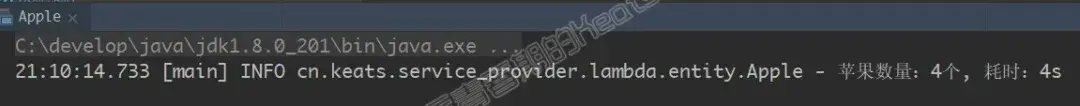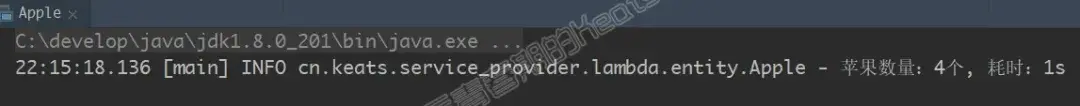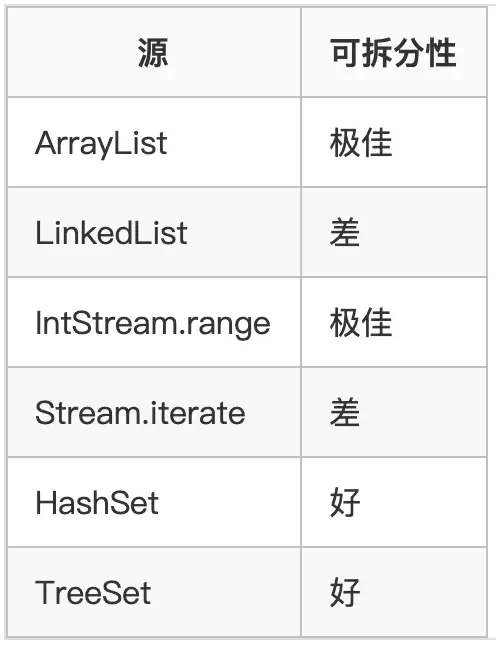1. 手动分成几部分

2. 为每部分创建线程

3. 在适当的时候合并

## 并行流

### 认识和开启并行流

``````List<Apple> appleList = new ArrayList<>(); // 假装数据是从库里查出来的

for (Apple apple : appleList) {
apple.setPrice(5.0 * apple.getWeight() / 1000);
}

``````

``````appleList.parallelStream().forEach(apple -> apple.setPrice(5.0 * apple.getWeight() / 1000));

``````

java.util.concurrent.ForkJoinPool.common.parallelism 可以改变线程池大小。不过该值是全局变量。改变他会影响所有并行流。目前还无法为每个流配置专属的线程数。一般来说采用处理器核心数是不错的选择

### 测试并行流的性能

``````public static void main(String[] args) throws InterruptedException {
List<Apple> appleList = initAppleList();

Date begin = new Date();
for (Apple apple : appleList) {
apple.setPrice(5.0 * apple.getWeight() / 1000);
Thread.sleep(1000);
}
Date end = new Date();
log.info("苹果数量：{}个, 耗时：{}s", appleList.size(), (end.getTime() - begin.getTime()) /1000);
}

``````### 并行版本

``````List<Apple> appleList = initAppleList();

Date begin = new Date();
appleList.parallelStream().forEach(apple ->
{
apple.setPrice(5.0 * apple.getWeight() / 1000);
try {
Thread.sleep(1000);
}catch (InterruptedException e) {
e.printStackTrace();
}
});
Date end = new Date();
log.info("苹果数量：{}个, 耗时：{}s", appleList.size(), (end.getTime() - begin.getTime()) /1000);

``````## 并行流可以随便用吗？

### 可拆分性影响流的速度

1. 对于 iterate 方法来处理的前 n 个数字来说，不管并行与否，它总是慢于循环的，非并行版本可以理解为流化操作没有循环更偏向底层导致的慢。可并行版本是为什么慢呢？这里有两个需要注意的点：

• iterate 生成的是装箱的对象，必须拆箱成数字才能求和

• 我们很难把 iterate 分成多个独立的块来并行执行

2. 而对于 LongStream.rangeClosed() 方法来说，就不存在 iterate 的第两个痛点了。它生成的是基本类型的值，不用拆装箱操作，另外它可以直接将要生成的数字 1 - n 拆分成 1 - n/4， 1n/4 - 2n/4， ... 3n/4 - n 这样四部分。因此并行状态下的 rangeClosed() 是快于 for 循环外部迭代的：

``````package lambdasinaction.chap7;

import java.util.stream.*;

public class ParallelStreams {

public static long iterativeSum(long n) {
long result = 0;
for (long i = 0; i <= n; i++) {
result += i;
}
return result;
}

public static long sequentialSum(long n) {
return Stream.iterate(1L, i -> i + 1).limit(n).reduce(Long::sum).get();
}

public static long parallelSum(long n) {
return Stream.iterate(1L, i -> i + 1).limit(n).parallel().reduce(Long::sum).get();
}

public static long rangedSum(long n) {
return LongStream.rangeClosed(1, n).reduce(Long::sum).getAsLong();
}

public static long parallelRangedSum(long n) {
return LongStream.rangeClosed(1, n).parallel().reduce(Long::sum).getAsLong();
}
}

package lambdasinaction.chap7;

import java.util.concurrent.*;
import java.util.function.*;

public class ParallelStreamsHarness {

public static final ForkJoinPool FORK_JOIN_POOL = new ForkJoinPool();

public static void main(String[] args) {
System.out.println("Iterative Sum done in: " + measurePerf(ParallelStreams::iterativeSum, 10_000_000L) + " msecs");
System.out.println("Sequential Sum done in: " + measurePerf(ParallelStreams::sequentialSum, 10_000_000L) + " msecs");
System.out.println("Parallel forkJoinSum done in: " + measurePerf(ParallelStreams::parallelSum, 10_000_000L) + " msecs" );
System.out.println("Range forkJoinSum done in: " + measurePerf(ParallelStreams::rangedSum, 10_000_000L) + " msecs");
System.out.println("Parallel range forkJoinSum done in: " + measurePerf(ParallelStreams::parallelRangedSum, 10_000_000L) + " msecs" );
}

public static <T, R> long measurePerf(Function<T, R> f, T input) {
long fastest = Long.MAX_VALUE;
for (int i = 0; i < 10; i++) {
long start = System.nanoTime();
R result = f.apply(input);
long duration = (System.nanoTime() - start) / 1_000_000;
System.out.println("Result: " + result);
if (duration < fastest) fastest = duration;
}
return fastest;
}
}

``````

### 共享变量修改的问题

``````public static long sideEffectSum(long n) {
Accumulator accumulator = new Accumulator();
LongStream.rangeClosed(1, n).forEach(accumulator::add);
return accumulator.total;
}

public static long sideEffectParallelSum(long n) {
Accumulator accumulator = new Accumulator();
LongStream.rangeClosed(1, n).parallel().forEach(accumulator::add);
return accumulator.total;
}

public static class Accumulator {
private long total = 0;

public void add(long value) {
total += value;
}
}

``````

### 并行流的使用注意

• 对于较少的数据量，不建议使用并行流

• 容易拆分成块的流数据，建议使用并行流

• 以下是一些常见的集合框架对应流的可拆分性能表Scroll to Top